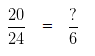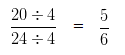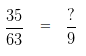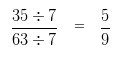# Finding Equivalent Fraction with given Denominator

Question based on Finding an Equivalent Fraction with given Denominator

Question 1

Write an equivalent Fraction of 20/24 with denominator 6 .

Explanation

Let,In order to obtain an equivalent fraction, we need to divide the Numerator and Denominator of a given Fraction, by the same digit

To get 6 in the denominator, we need to divide 24 by 4

So, in order to get an equivalent Fraction, we have to divide the Numerator also by 4

i.e,Hence, 5/6 is an equivalent Fraction of 20/24 with denominator 6

Question 2

Write an equivalent Fraction of 35/63 with denominator 9 .

Explanation

Let,In order to obtain an equivalent fraction, we need to divide the Numerator and Denominator of a given Fraction, by the same digit

To get 9 in the denominator, we need to divide 63 by 7

So, in order to get an equivalent Fraction, we have to divide the  Numerator also by 7

i.e,Hence, 5/9 is an equivalent Fraction of 35/63 with denominator 9